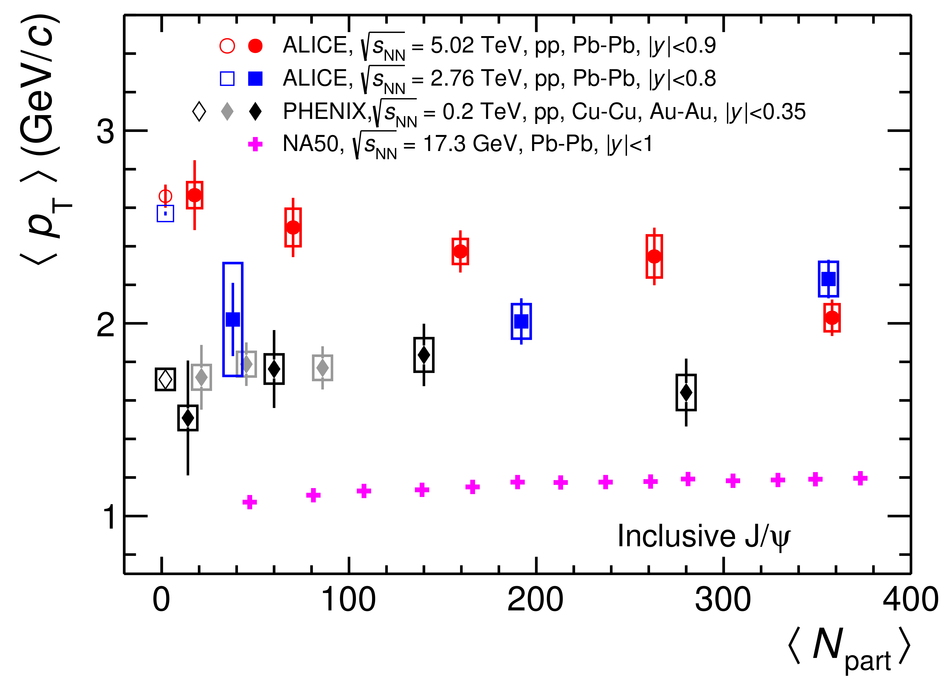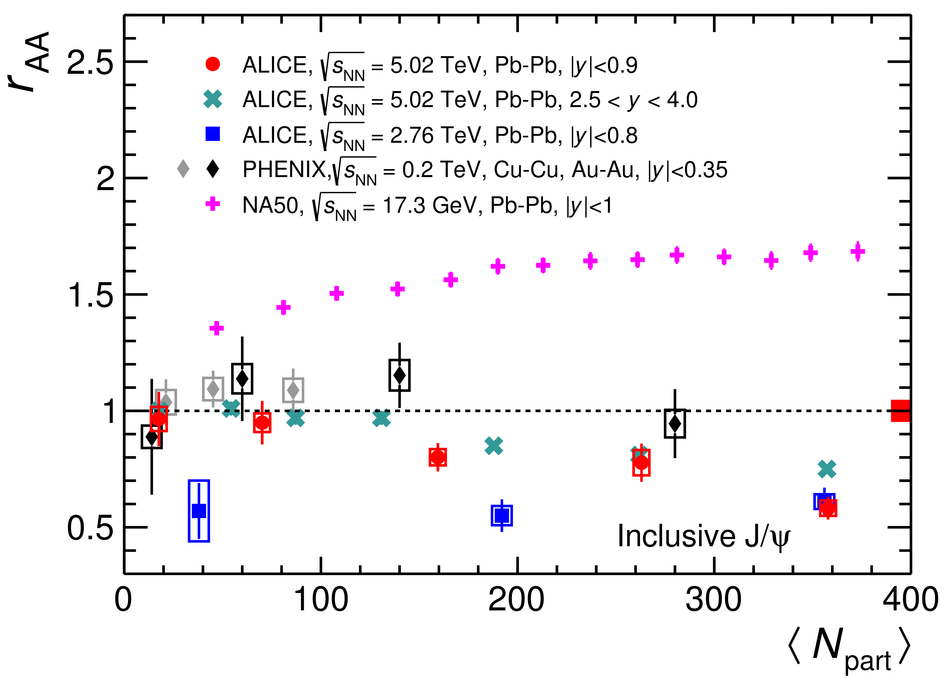# Figure 4

 $\jpsi$ $\MeanPt$ (left) and $\raa$ (right) at midrapidity as a function of the mean number of participant nucleons $\AvgNpart$. The ALICE measurements at $\snn = 5.02$ TeV are compared with previous results in $\pp$ and $\PbPb$ collisions at $2.76$ TeV , $\PbPb$ collisions at $5.02$ at forward rapidity , and with those at lower collision energies at SPS  and RHIC . The red box around unity at $\Npart \approx 400$ in the right panel indicates the correlated uncertainty of the ALICE data points due to the $\MeanPtSq$ in $\pp$ collisions.